GeeksforGeeks App
Open AppBrowser
Continue

# Class 10 NCERT Solutions- Chapter 7 Coordinate Geometry – Exercise 7.3

### (i) (2, 3), (-1, 0), (2, -4)

Solution:

Area = 1/2 [x1(y2 – y3) + x2(y3 – y1) + x3(y1 – y2)]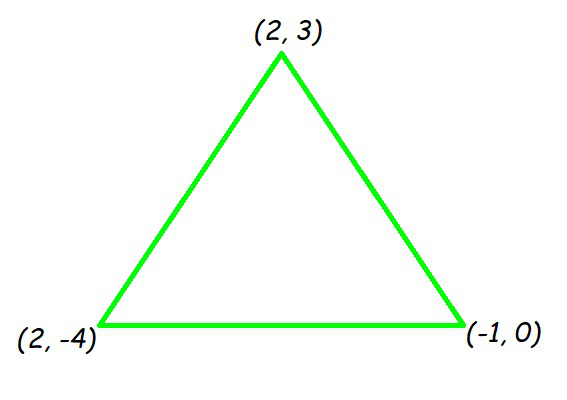Now by putting all the values in the formula, we will get

Area of triangle = 1/2 [2(0 – (-4)) + (-1)((-4) – (3)) + 2(3 – 0)]

= 1/2 [8 + 7 + 6]

= 21/2

So, the area of triangle is 21/2 square units.

### (ii) (-5, -1), (3, -5), (5, 2)

Solution:

Area = 1/2 [x1(y2 – y3) + x2(y3 – y1) + x3(y1 – y2)]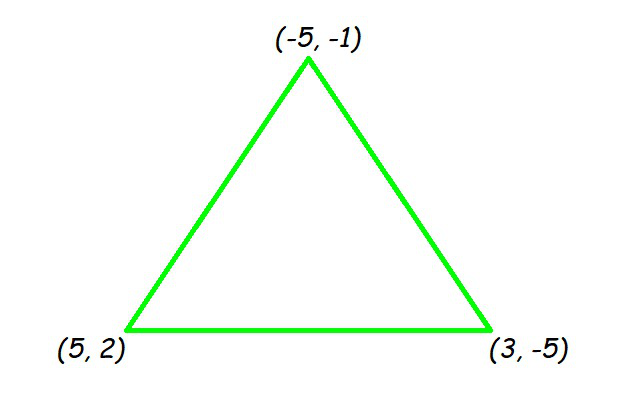Area of the triangle = 1/2 [(-5)((-5) – (2)) + 3(2 – (-1)) + 5((-1) – (-5))]

= 1/2[35 + 9 + 20]

= 32

So, the area of the triangle is 32 square units.

### (i) (7, -2), (5, 1), (3, -k)

Solution:

As we know the result, for collinear points, area of triangle formed by them is always zero.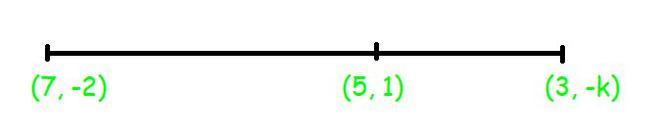Let points (7, -2) (5, 1), and (3, k) are vertices of a triangle. (As given in the question)

Area of triangle = 1/2 [7(1 – k) + 5(k – (-2)) + 3((-2) – 1)] = 0

7 – 7k + 5k +10 -9 = 0

-2k + 8 = 0

k = 4

### (ii) (8, 1), (k, -4), (2, -5)

Solution:

As we know the result, for collinear points, area of triangle formed by them is zero.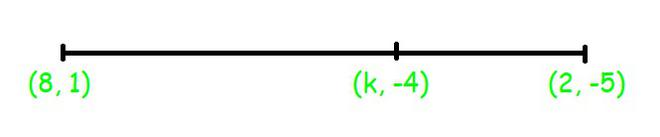So we can say that for points (8, 1), (k, – 4), and (2, – 5), area = 0

1/2 [8((-4) – (-5)) + k((-5) – (1)) + 2(1 -(-4))] = 0

8 – 6k + 10 = 0

6k = 18

k = 3

### Question 3. Find the area of the triangle formed by joining the mid-points of the sides of the triangle whose vertices are (0, -1), (2, 1), and (0, 3). Find the ratio of this area to the area of the given triangle.

Solution:

Let us assume that vertices of the triangle be A(0, -1), B(2, 1), C(0, 3).

Let us assume that D, E, F be the mid-points of the sides of triangle.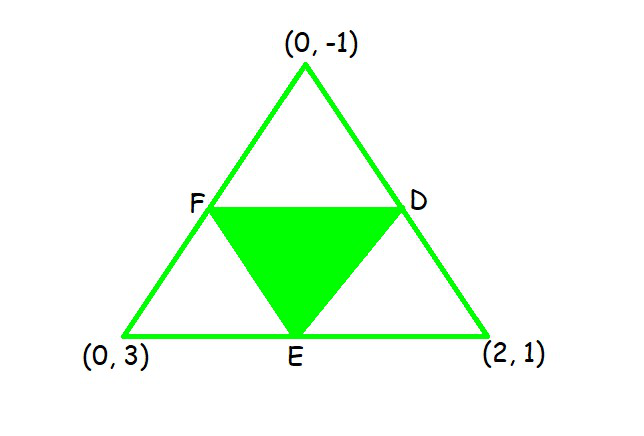Coordinates of D, E, and F are

D = ((0 + 2)/2, (-1 + 1)/2) = (1, 0)

E = ((0+ 0)/2, (-1 + 3)/2) = (0, 1)

F = ((2+0)/2, (1 + 3)/2) = (1, 2)

Area(ΔDEF) = 1/2 [1(2 – 1) + 1(1 – 0) + 0(0 – 2)]  = 1/2 (1+1) = 1

Area of ΔDEF is 1 square units

Area(ΔABC) = 1/2 [0(1 – 3) + 2(3 – (-1)) + 0((-1) – 1)] = 1/2  = 4

Area of ΔABC is 4 square units

So, the required ratio is 1:4.

### Question 4. Find the area of the quadrilateral whose vertices, taken in order, are (-4, -2), (-3, -5), (3, -2), and (2, 3).

Solution:

Let the vertices of the quadrilateral be A(-4, -2), B(-3, -5), C(3, -2), and D(2, 3).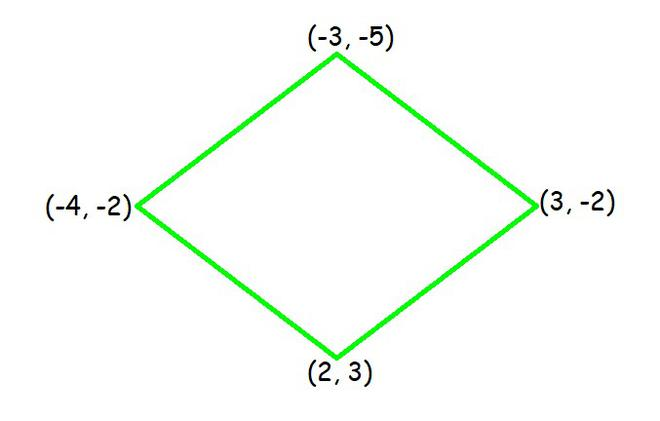Here, AC divide quadrilateral into two triangles.

Now, we have two triangles ΔABC and ΔACD.

Area of ΔABC = 1/2 [(-4)((-5) – (-2)) + (-3)((-2) – (-2)) + 3((-2) – (-5))]

= 1/2 [12 + 0 + 9]

= 21/2 square units

Area of ΔACD = 1/2 [(-4)((-2) – (3)) + 3((3) – (-2)) + 2((-2) – (-2))]

= 1/2 [20 + 15 + 0]

= 35/2 square units

Now we will add area of both triangle and resultant will give area of quadrilateral

Area of quadrilateral ABCD = Area of ΔABC + Area of ΔACD

= (21/2 + 35/2) square units = 28 square units

### Question 5. You have studied in Class IX that a median of a triangle divides it into two triangles of equal areas. Verify this result for ΔABC whose vertices are A (4, -6), B (3, -2), and C (5, 2).

Solution:

Let the vertices of the triangle be A(4, -6), B(3, -2), and C(5, 2).

Let us assume that D be the mid-point of side BC of ΔABC. So, AD is the median in ΔABC.

Coordinates of point D = Midpoint of BC = (4, 0)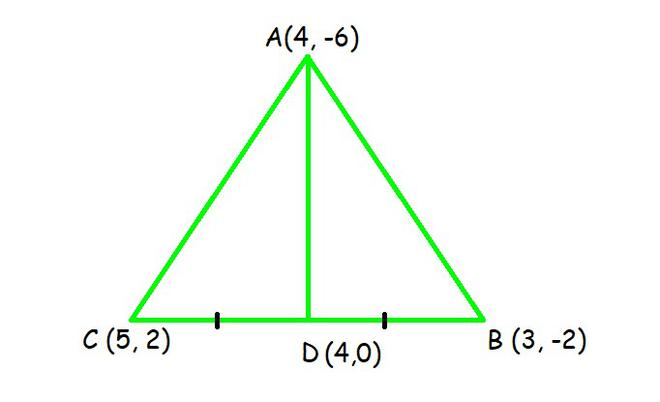Formula: Area = 1/2 [x1(y2 – y3) + x2(y3 – y1) + x3(y1 – y2)]

Now,

Area of ΔABD = 1/2 [(4)((-2) – (0)) + 3((0) – (-6)) + (4)((-6) – (-2))]

= 1/2 [-8 + 18 – 16]

= -3 square units

As we know that, area cannot be negative. So, the area of ΔABD is 3 square units.

Area of ΔACD = 1/2 [(4)(0 – (2)) + 4((2) – (-6)) + (5)((-6) – (0))]

= 1/2 [-8 + 32 – 30]

= -3 square units

As we know that, area cannot be negative. So, the area of ΔACD is 3 square units.

The area of both sides is same.

So we can say that, median AD has divided ΔABC in two triangles of equal areas.

My Personal Notes arrow_drop_up
Related Tutorials